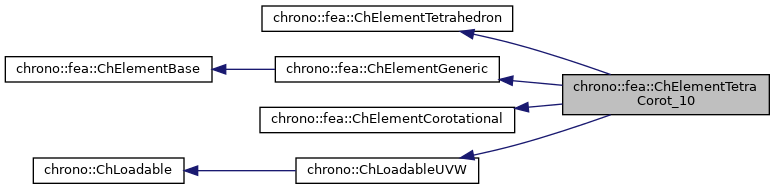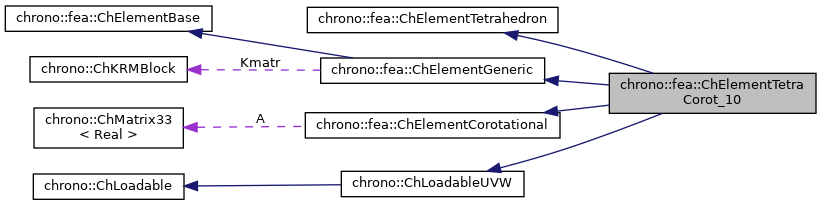chrono::fea::ChElementTetraCorot_10 Class Reference

## Description

Tetrahedron FEA element with 10 nodes.

This is a quadratic element for displacements; stress and strain are interpolated depending on Gauss points.

#include <ChElementTetraCorot_10.h>

Inheritance diagram for chrono::fea::ChElementTetraCorot_10:[legend]
Collaboration diagram for chrono::fea::ChElementTetraCorot_10:[legend]

## Public Types

using ShapeVector = ChMatrixNM< double, 1, 10 >

## Public Member Functions

virtual int GetNnodes () override
Get the number of nodes used by this element.

virtual int GetNdofs () override
Get the number of coordinates in the field used by the referenced nodes. More...

virtual int GetNodeNdofs (int n) override
Get the number of coordinates from the specified node that are used by this element. More...

double GetVolume ()

virtual std::shared_ptr< ChNodeFEAbaseGetNodeN (int n) override
Access the nth node.

virtual std::shared_ptr< ChNodeFEAxyzGetTetrahedronNode (int n) override
Return the specified tetrahedron node (0 <= n <= 3).

virtual void SetNodes (std::shared_ptr< ChNodeFEAxyz > nodeA, std::shared_ptr< ChNodeFEAxyz > nodeB, std::shared_ptr< ChNodeFEAxyz > nodeC, std::shared_ptr< ChNodeFEAxyz > nodeD, std::shared_ptr< ChNodeFEAxyz > nodeE, std::shared_ptr< ChNodeFEAxyz > nodeF, std::shared_ptr< ChNodeFEAxyz > nodeG, std::shared_ptr< ChNodeFEAxyz > nodeH, std::shared_ptr< ChNodeFEAxyz > nodeI, std::shared_ptr< ChNodeFEAxyz > nodeJ)

virtual void Update () override
Update element at each time step.

void ShapeFunctions (ShapeVector &N, double r, double s, double t)
Fills the N shape function matrix with the values of shape functions at zi parametric coordinates, where r=1 at 2nd vertex, s=1 at 3rd, t=1 at 4th. More...

virtual void GetStateBlock (ChVectorDynamic<> &mD) override
Fills the D vector (displacement) with the current field values at the nodes of the element, with proper ordering. More...

double ComputeVolume ()
Approximation!! not the exact volume This returns an exact value only in case of Constant Metric Tetrahedron.

virtual void ComputeJacobian (ChMatrixDynamic<> &Jacobian, double zeta1, double zeta2, double zeta3, double zeta4)
Puts inside 'Jacobian' the Jacobian matrix of the element zeta1,...,zeta4 are the four natural coordinates of the integration point note: in case of tetrahedral elements natural coord. More...

virtual void ComputeMatrB (ChMatrixDynamic<> &mmatrB, double zeta1, double zeta2, double zeta3, double zeta4, double &JacobianDet)
Computes the matrix of partial derivatives and puts data in "mmatrB" evaluated at natural coordinates zeta1,...,zeta4 note: in case of tetrahedral elements natural coord. More...

virtual void ComputeStiffnessMatrix ()
Computes the local STIFFNESS MATRIX of the element: K = sum (w_i * [B]' * [D] * [B]) More...

void GetParameterForNodeID (const int nodeID, double &z1, double &z2, double &z3, double &z4)
Given the node ID, gets the 4 parameters of the shape function.

ChStrainTensor GetStrain (double z1, double z2, double z3, double z4)
Returns the strain tensor at given parameters. More...

ChStressTensor GetStress (double z1, double z2, double z3, double z4)
Returns the stress tensor at given parameters. More...

virtual void UpdateRotation () override
Given the actual position of the nodes, recompute the cumulative rotation matrix A.

virtual void ComputeKRMmatricesGlobal (ChMatrixRef H, double Kfactor, double Rfactor=0, double Mfactor=0) override
Sets H as the global stiffness matrix K, scaled by Kfactor. More...

virtual void ComputeInternalForces (ChVectorDynamic<> &Fi) override
Computes the internal forces (ex. More...

void SetMaterial (std::shared_ptr< ChContinuumElastic > my_material)
Set the material of the element.

std::shared_ptr< ChContinuumElasticGetMaterial ()

const ChMatrixDynamicGetMatrB (int n) const
Get the partial derivatives matrix MatrB and the StiffnessMatrix.

const ChMatrixDynamicGetStiffnessMatrix () const

Gets the number of DOFs affected by this element (position part)

Gets the number of DOFs affected by this element (speed part)

virtual void LoadableGetStateBlock_x (int block_offset, ChState &mD) override
Gets all the DOFs packed in a single vector (position part)

virtual void LoadableGetStateBlock_w (int block_offset, ChStateDelta &mD) override
Gets all the DOFs packed in a single vector (speed part)

virtual void LoadableStateIncrement (const unsigned int off_x, ChState &x_new, const ChState &x, const unsigned int off_v, const ChStateDelta &Dv) override
Increment all DOFs using a delta.

virtual int Get_field_ncoords () override
Number of coordinates in the interpolated field: here the {x,y,z} displacement.

virtual int GetSubBlocks () override
Get the number of DOFs sub-blocks.

virtual unsigned int GetSubBlockOffset (int nblock) override
Get the offset of the specified sub-block of DOFs in global vector.

virtual unsigned int GetSubBlockSize (int nblock) override
Get the size of the specified sub-block of DOFs in global vector.

virtual bool IsSubBlockActive (int nblock) const override
Check if the specified sub-block of DOFs is active.

virtual void LoadableGetVariables (std::vector< ChVariables * > &mvars) override
Get the pointers to the contained ChVariables, appending to the mvars vector.

virtual void ComputeNF (const double U, const double V, const double W, ChVectorDynamic<> &Qi, double &detJ, const ChVectorDynamic<> &F, ChVectorDynamic<> *state_x, ChVectorDynamic<> *state_w) override
Evaluate N'*F , where N is some type of shape function evaluated at U,V,W coordinates of the volume, each ranging in -1..+1 F is a load, N'*F is the resulting generalized load Returns also det[J] with J=[dx/du,..], that might be useful in gauss quadrature. More...

virtual double GetDensity () override
This is needed so that it can be accessed by ChLoaderVolumeGravity.

virtual bool IsTetrahedronIntegrationNeeded () override
If true, use quadrature over u,v,w in [0..1] range as tetrahedron volumetric coords, with z=1-u-v-w otherwise use quadrature over u,v,w in [-1..+1] as box isoparametric coords.Public Member Functions inherited from chrono::fea::ChElementGeneric
ChKblockGenericKstiffness ()
Access the proxy to stiffness, for sparse solver.

virtual void EleIntLoadResidual_F (ChVectorDynamic<> &R, const double c) override
Add the internal forces (pasted at global nodes offsets) into a global vector R, multiplied by a scaling factor c, as R += forces * c This default implementation is SLIGHTLY INEFFICIENT.

virtual void EleIntLoadResidual_Mv (ChVectorDynamic<> &R, const ChVectorDynamic<> &w, const double c) override
Add the product of element mass M by a vector w (pasted at global nodes offsets) into a global vector R, multiplied by a scaling factor c, as R += M * w * c This default implementation is VERY INEFFICIENT.

virtual void EleIntLoadResidual_F_gravity (ChVectorDynamic<> &R, const ChVector<> &G_acc, const double c) override
Add the contribution of gravity loads, multiplied by a scaling factor c, as: R += M * g * c This default implementation is VERY INEFFICIENT. More...

virtual void ComputeGravityForces (ChVectorDynamic<> &Fg, const ChVector<> &G_acc) override
Compute the gravitational forces. More...

virtual void ComputeMmatrixGlobal (ChMatrixRef M) override
Calculate the mass matrix, expressed in global reference. More...

virtual void InjectKRMmatrices (ChSystemDescriptor &descriptor) override
Tell to a system descriptor that there are item(s) of type ChKblock in this object (for further passing it to a solver)

virtual void KRMmatricesLoad (double Kfactor, double Rfactor, double Mfactor) override
Add the current stiffness K and damping R and mass M matrices in encapsulated ChKblock item(s), if any. More...

virtual void VariablesFbLoadInternalForces (double factor=1.) override
Add the internal forces, expressed as nodal forces, into the encapsulated ChVariables.

virtual void VariablesFbIncrementMq () override
Add M*q (internal masses multiplied current 'qb').Public Member Functions inherited from chrono::fea::ChElementBase
virtual int GetNdofs_active ()
Get the actual number of active degrees of freedom. More...

virtual int GetNodeNdofs_active (int n)
Get the actual number of active coordinates from the specified node that are used by this element. More...

virtual void ComputeNodalMass ()
Compute element's nodal masses.

virtual void EleDoIntegration ()
This is optionally implemented if there is some internal state that requires integration.Public Member Functions inherited from chrono::fea::ChElementCorotational
ChMatrix33Rotation ()
Access the cumulative rotation matrix of the element. More...Public Member Functions inherited from chrono::ChLoadableUVW
virtual bool IsTrianglePrismIntegrationNeeded ()
If true, use quadrature over u,v in [0..1] range as triangle natural coords (with z=1-u-v), and use linear quadrature over w in [-1..+1], otherwise use default quadrature over u,v,w in [-1..+1] as box isoparametric coords.Protected Attributes inherited from chrono::fea::ChElementGeneric
ChKblockGeneric KmatrProtected Attributes inherited from chrono::fea::ChElementCorotational
ChMatrix33 A

## ◆ ComputeInternalForces()

 void chrono::fea::ChElementTetraCorot_10::ComputeInternalForces ( ChVectorDynamic<> & Fi )
overridevirtual

Computes the internal forces (ex.

the actual position of nodes is not in relaxed reference position) and set values in the Fi vector.

Implements chrono::fea::ChElementBase.

## ◆ ComputeJacobian()

 void chrono::fea::ChElementTetraCorot_10::ComputeJacobian ( ChMatrixDynamic<> & Jacobian, double zeta1, double zeta2, double zeta3, double zeta4 )
virtual

Puts inside 'Jacobian' the Jacobian matrix of the element zeta1,...,zeta4 are the four natural coordinates of the integration point note: in case of tetrahedral elements natural coord.

vary in the range 0 ... +1

## ◆ ComputeKRMmatricesGlobal()

 void chrono::fea::ChElementTetraCorot_10::ComputeKRMmatricesGlobal ( ChMatrixRef H, double Kfactor, double Rfactor = 0, double Mfactor = 0 )
overridevirtual

Sets H as the global stiffness matrix K, scaled by Kfactor.

Optionally, also superimposes global damping matrix R, scaled by Rfactor, and global mass matrix M multiplied by Mfactor.

Implements chrono::fea::ChElementBase.

## ◆ ComputeMatrB()

 void chrono::fea::ChElementTetraCorot_10::ComputeMatrB ( ChMatrixDynamic<> & mmatrB, double zeta1, double zeta2, double zeta3, double zeta4, double & JacobianDet )
virtual

Computes the matrix of partial derivatives and puts data in "mmatrB" evaluated at natural coordinates zeta1,...,zeta4 note: in case of tetrahedral elements natural coord.

vary in the range 0 ... +1

## ◆ ComputeNF()

 void chrono::fea::ChElementTetraCorot_10::ComputeNF ( const double U, const double V, const double W, ChVectorDynamic<> & Qi, double & detJ, const ChVectorDynamic<> & F, ChVectorDynamic<> * state_x, ChVectorDynamic<> * state_w )
overridevirtual

Evaluate N'*F , where N is some type of shape function evaluated at U,V,W coordinates of the volume, each ranging in -1..+1 F is a load, N'*F is the resulting generalized load Returns also det[J] with J=[dx/du,..], that might be useful in gauss quadrature.

Parameters
 U parametric coordinate in volume V parametric coordinate in volume W parametric coordinate in volume Qi Return result of N'*F here, maybe with offset block_offset detJ Return det[J] here F Input F vector, size is = n.field coords. state_x if != 0, update state (pos. part) to this, then evaluate Q state_w if != 0, update state (speed part) to this, then evaluate Q

## ◆ ComputeStiffnessMatrix()

 void chrono::fea::ChElementTetraCorot_10::ComputeStiffnessMatrix ( )
virtual

Computes the local STIFFNESS MATRIX of the element: K = sum (w_i * [B]' * [D] * [B])

!! => because the canonical interval is -1 ... +1, but we want to integrate

## ◆ GetNdofs()

 virtual int chrono::fea::ChElementTetraCorot_10::GetNdofs ( )
inlineoverridevirtual

Get the number of coordinates in the field used by the referenced nodes.

This is for example the size (number of rows/columns) of the local stiffness matrix.

Implements chrono::fea::ChElementBase.

## ◆ GetNodeNdofs()

 virtual int chrono::fea::ChElementTetraCorot_10::GetNodeNdofs ( int n )
inlineoverridevirtual

Get the number of coordinates from the specified node that are used by this element.

Note that this may be different from the value returned by GetNodeN(n)->GetNdofW().

Implements chrono::fea::ChElementBase.

## ◆ GetStateBlock()

 void chrono::fea::ChElementTetraCorot_10::GetStateBlock ( ChVectorDynamic<> & mD )
overridevirtual

Fills the D vector (displacement) with the current field values at the nodes of the element, with proper ordering.

If the D vector has not the size of this->GetNdofs(), it will be resized. For corotational elements, field is assumed in local reference!

Implements chrono::fea::ChElementBase.

## ◆ GetStrain()

 ChStrainTensor chrono::fea::ChElementTetraCorot_10::GetStrain ( double z1, double z2, double z3, double z4 )

Returns the strain tensor at given parameters.

The tensor is in the original undeformed unrotated reference.

## ◆ GetStress()

 ChStressTensor chrono::fea::ChElementTetraCorot_10::GetStress ( double z1, double z2, double z3, double z4 )

Returns the stress tensor at given parameters.

The tensor is in the original undeformed unrotated reference.

## ◆ ShapeFunctions()

 void chrono::fea::ChElementTetraCorot_10::ShapeFunctions ( ShapeVector & N, double r, double s, double t )

Fills the N shape function matrix with the values of shape functions at zi parametric coordinates, where r=1 at 2nd vertex, s=1 at 3rd, t=1 at 4th.

All ranging in [0...1]. The last, u (=1 at 1st vertex) is computed form the first 3. It stores the Ni(r,s,t) values in a 1 row, 10 columns matrix.

The documentation for this class was generated from the following files:
• /builds/uwsbel/chrono/src/chrono/fea/ChElementTetraCorot_10.h
• /builds/uwsbel/chrono/src/chrono/fea/ChElementTetraCorot_10.cpp